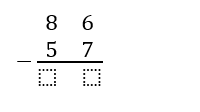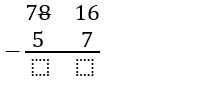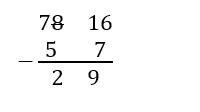# Two Digit Subtraction Worksheets

Tips for Double Digit Subtraction When you are teaching young minds about the concept of subtraction, moving onto double digit subtraction is a giant leap forward. However, this is also the time when they will be able to handle such division practices with ease. Therefore, in order to ensure that the students will be able to resolve double digit subtraction easily is by following the tips given below. Firstly, make them learn the concepts of place values. This way, they will be able to understand the concept of borrowing numbers. Now, let us consider an example of 86 – 57. Now, let us solve the question in a sequential manner.Now, the first step is the students must keep in mind that only a larger number can subtract a smaller one and not vice versa. Therefore, in the scenario, the once a digit is 6 – 7 which is not possible. Therefore, we must borrow 1 from 8 to make 6 into 16.Now, we can perform subtraction.• ### Basic Lesson

Demonstrates subtraction from the ones and tens columns.

• ### Intermediate Lesson

This lesson demonstrates how to borrow between ones and tens columns.

• ### Independent Practice 1

Contains 20 two digit subtraction problems. The answers can be found below.

• ### Independent Practice 2

Another 20 practice problems. The answer key is below.

• ### Homework Worksheet

Reviews the skills within the unit and provides 12 practice problems.

• ### Skill Quiz

10 two digit subtraction math problems to help assess the unit. A math scoring matrix is included.

• ### Homework and Quiz Answer Key

Answers for the homework and quiz.